﻿ Interpolation of Climatic Parameters By Using Barycentric Coordinates

### Interpolation of Climatic Parameters By Using Barycentric Coordinates

Khurshidbek MAKHMUDOV, Yasuhiro MITANI, Tetsuya KUSUDAOPEN ACCESSPEER-REVIEWED

## Interpolation of Climatic Parameters By Using Barycentric Coordinates

Khurshidbek MAKHMUDOV1,, Yasuhiro MITANI1, Tetsuya KUSUDA1

1Department of Civil Engineering, Graduate School of Engineering, Kyushu Univ., 774 Motooka, Nishi-ku, Fukuoka 819-0395 JAPAN

### Abstract

In this paper, we propose a new method of interpolation of climatic parameters territory spread values point measurements of meteorological data on the territories of its space. The method is based on the representation of territories affected by hydro-meteorological stations as the mechanical integrity of the system, consisting of a set of material particles having a common centre of motion. An example of such a centre is known in practice barycenter (center of pressure). Similarity features of change in the value space of meteorological parameters:pressure, rainfall, humidity and other parameters allowed us to develop an interpolation method, based on the well -known method of classical mechanics, a method for finding centers systems. Centers Systems: barycenter, center humidity, precipitation, etc., are reference points from which climate data set gradients (gradient is a vector indicating the direction of change of a scalar quantity. The pressure, rainfall, temperature and humidity are known as scalars). Gradients and equation are describing the basis methods for the proposed interpolation. Graphical method of calculations carried applicability straight-line equation for the interpolation of climate data. The method is entirely based on the use of proven analytical dependences and therefore reliable results. Calculations are made with specific parameters for the territory of the proposed method and the results of their tests are set to points to the checked area. Humidity value for the calculated period (average statistical value according to meteorological stations) is taken 72.25%, while the real value according to the results of our estimations is 59.34%. In this instance the climate accuracy value is used by 17.4%. For the atmospheric pressure value, error currently used in pressure standards which is estimated (assessed) during this period is 5.7%. Our proposed method contributes with sufficiently high accuracy set the values of the climatic parameters of the territory at any point.

### At a glance: Figures

12
Prev Next

• MAKHMUDOV, Khurshidbek, Yasuhiro MITANI, and Tetsuya KUSUDA. "Interpolation of Climatic Parameters By Using Barycentric Coordinates." World Journal of Environmental Engineering 3.1 (2015): 1-6.
• MAKHMUDOV, K. , MITANI, Y. , & KUSUDA, T. (2015). Interpolation of Climatic Parameters By Using Barycentric Coordinates. World Journal of Environmental Engineering, 3(1), 1-6.
• MAKHMUDOV, Khurshidbek, Yasuhiro MITANI, and Tetsuya KUSUDA. "Interpolation of Climatic Parameters By Using Barycentric Coordinates." World Journal of Environmental Engineering 3, no. 1 (2015): 1-6.

 Import into BibTeX Import into EndNote Import into RefMan Import into RefWorks

### 1. Introduction

Planning and management of water for irrigation in the Arid Areas implemented depending on the type of plants on the basis of their mode of irrigation. Thus management of water for irrigation is performed using meteorological data areas: atmospheric pressure, precipitation, evaporation, humidity areas, etc. Currently meteorological data areas are established through point measurements on specially equipped weather stations. Each weather station has a coverage radius that ranges 15-35 km and more. Meanwhile meteorological parameters territories are heavily dependent on the terrain and crossing an area meteorological parameter values may undergo significant changes in the area. Use not sufficiently accurate weather information reduces the efficiency of the ground - water and a negative impact on crop yields. And coverage of the entire territory of the necessary number of weather stations from both a technical and economic point so view is not possible. Therefore, various methods of interpolation of climate data, which are used as a basis for weather data. A variety of methods known interpolation areas, the most common of these are: the method of inverse distance weighted method kriging and trend surface . The method points which are closer to those, in which the assessment is made, have a greater impact as compared to distant points. For a more accurate description of the topography of a set of points, which will be interpolated, chosen in a neighborhood defined point, as they have the greatest influence on her height is based on the reduction of the required point lying near to the reference point. The method is the basis for determining the radius of influence of meteorological stations.

In the method of trend surface, a set of points within a given neighborhood. Within each neighborhood built surface of best approximation based on mathematical equations, such as polynomials or splines. For this purpose usually the least squares. These equations are described by nonlinear dependencies, replacing curves or other forms of numerical sequences into simpler. To construct the surface of the trend in each value is substituted into equation vicinity. As a result, we obtain one value that is assigned to the interpolated point. For other target points continues this process.

Common method of interpolation is natural factors territories Kriging . It involves the use of spatial continuity model or relationship (in the form of covariance - scalable version which is semivariogram correlation or model landscape site), and the sample surface data to determine trends, statistics on which holds interpolation ( extrapolation ) of the points. Quantitative representation of spatial data structures, known as building variogram or semivariogram, enables users to choose the data model of spatial dependence. To calculate the (forecast) of the unknown value of a variable at a given point kriging will use the appropriate (matched) model variograms, spatial data and configuration values in the measurement points around the location.

Also used cokriging - this interpolation method which allows to calculate the surface cubes and cards using the statistics for multiple datasets that this method of interpolation adds additional correlation between the data analysis capabilities.

Above mentioned interpolation methods are based on the original data of the reference station and a reference station while may not reflect all features of the basin. On the example of Chirchik river basin area, weather station, which would characterize the entire territory of features not. And the transition from zone to another meteorological parameters undergo serious changes. In connection with this method of inverse distance weighted method and the surface of the trend were not used.

Widespread methods of kriging and co-kriging, has great opportunities, in particular for the use of GIS technology, but they are very complicated and time-consuming. Kriging and cokriging methods based on natural features of the territory is more useful to describe the landscapes and their characteristics. A meteorological data in addition to the natural features of the territories are also related spatial arrangement of interpolated parameters territories. The complexity and the complexity of the kriging and cokriging methods primarily due to the need to create variograms and semivariogram that dramatically different landscape areas arid and semiarid zones, which in particular is Chirchik river basin is not possible.

In this regard, the proposed interpolation method became necessary to create a combined system consisting of a landscape, and the natural component of a system of point system of a moving mass, with a single center. In this territory meteorological components are accepted as elements of mass system having their centers of motion.

### 2. Materials and Methods

The object of investigation adopted Chirchik river basin area in the Republic of Uzbekistan. In this territory to monitor hydro-meteorological factors installed and operated four meteorological stations (Figure 1).

Select Location hydrometeorological stations in the basin ChirchikRiver caused primarily of natural and climatic conditions of the area and zone. Hydrometeorological Station Tashkent is located in central Tashkent and is naturally significantly influenced by anthropogenic factors. Hydrometeorological Station Chimghan is already on the mountainous part of the basin, where anthropogenic factors not significantly affect the natural processes. Hydrometeorological Station Yangiyo'l is on the flat part of the basin, where anthropogenic significant compared with the mountain area. On the territory of irrigated agriculture, for which reliable meteorological parameters play an important role for the planning and management of water for irrigation. Hydro-meteorological Station 4, located about Tuyabuguz reservoir, the area of the transition zone of the mountain landscape of the plains, here developed rain fed and irrigated agriculture. On rainfed cultivated mainly winter wheat and viticulture, the state of which is wholly dependent on climatic factors, primarily from precipitation. Although meteorological stations located in the most characteristic areas of the basin with matching radii coverage, but the methods Applicability interpolation methods do not allow to obtain reliable meteorological information is very necessary for a particular area of ​​the basin.

Our proposed new interpolation method based hydro-meteorological data territories, especially the consideration of the pool area as a system consisting of material points and is in a single movement and the whole in a single space. For such a system is the center point of the material, which characterizes the motion of the whole system, if applied pressure is a point called the barycenter or the center of the system. So other meteorological parameters such as temperature, humidity, precipitation, etc. are also elements of the system and their status changed identically as atmospheric pressure. To establish the center of the system or the center of hydrometeorological parameters for the start of the report - origin adopted weather station on the territory of Tashkent (Figure 1).

Figure 1. Layout of hydro-meteorological stations in the Chirchik river basin in Uzbekistan

As raw materials, research on the proposed method of taking the actual parameters of the basin Chirchik river, while to validate the results of the study used monthly averages for two months observing the different seasons in 2012 (Table 1, Table 2, Table 3, Table 4. Uzhydromet Source).

#### Table 1.

Values of the coordinates of hydro-meteorological stations positioned relative origin Tashkent weather station in meters. To establish the coordinates of the center of pressure and other meteorological parameters (the point at which the average set of meteorological parameters) as the origin or as a reference point also agreed location meteorological stations of Tashkent used dependence :(1)

Where- the radius vector of the center of mass,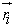- radius vector of the i -th point of the system,the mass of the i -th point. Then the coordinates of the center system (mass) can be rewritten as follows:

For pressure coordinates of the center of pressure: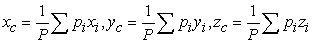(2)

Where the P -sum monthly averages of atmospheric pressure (Pi) for 4 meteorological stations

• For temperature- Т0С, coordinates of the center:(3)

Where Т is the sum of mean monthly temperature (Ti) air for 4 meteorological stations

For precipitation-O, the centre coordinates: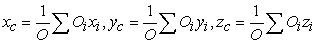(4)

Where О- is the sum of the average monthly precipitation (Oi ) for 4 meteorological stations

For humidity, the centre coordinates of humidity, where the humidity is equal to its average value: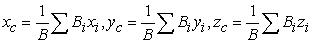(5)

Where, В - is the amount of average monthly humidity (Bi) for 4 meteorological stations xi, yi , zi -coordinates of hydrometeorological stations relative to the origin (station Tashkent).

The main part of the research

Using data of Uzhydromet (Table 1, Table 2, Table 3, Table 4) and equation (2-5) produce estimates coordinates of the centers (mass) of meteorological parameters in area (system) of Chirchik river basin. We have got the results as:

The average value of moisture %, corresponding to the center of mass is equal to 44,25%.

#### Table 5.

Thus, as a test case using monthly averages (data Uzhydromet) in February and June 2012 received the center of mass for atmospheric pressure and humidity at which the atmospheric pressure and humidity, respectively, are equal to their average value. Conducted test calculations have shown that the difference of meteorological parameters influence the position of their centres, each parameter: barometric pressure, rainfall, humidity and temperature, etc. has its centre. When this variability values of meteorological parameters in time also affects the position of their centers. In this regard, the proposed method for each meteorological parameter for a point in time (when the measurements) calculations must be performed according to this procedure separately. Table 2 gives examples of the results of calculations for two parameters: for atmospheric pressure and humidity, respectively, for two months of monthly data of different seasons in 2012. As the table shows the values of coordinates of the centres of pressure and humidity and different, at the same time they also differ on estimates of months.

Connecting the centre of mass with hydro obtain points (gradients) vectors showing the direction of change of meteorological parameters or gradients of atmospheric pressure and humidity.

For spatial interpolation of meteorological parameters are parallel transport coordinate system from the initial point "Tashkent meteo" on the centre of mass -C. Parallel transport coordinate system to atmospheric pressure is shown in Figure 2. Establish the coordinates of points of hydro-meteorological stations relatively new coordinate system with the origin - C were performed using known relationships of course analytic geometry: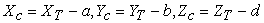(6)

Using (6) are calculated with respect to the coordinate values of weather-origin C and the corresponding monthly average meteorological parameters. In particular, the coordinates meteorological station "Chimghanmeteo" atmospheric pressure (in meters) measured in relation to monthly averages for February 2012 are Pch(51766,49;32088,03;1011,29).

Figure 2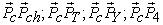pressure gradients.

Parallel transport coordinate system for humidity is shown in Figure 3. Calculated values of the coordinates are of all the weather stations in the basin, relative to the centre of humidity of Chirchik river system. In particular coordinate meteorological station Chimghan relative humidity center (system) areas (in meters) Sun-point with respect to the measured monthly averages for February 2012 are Bch (55947,77; 35110,47; 1089,82).

Figure 3- gradients of humidity.

Figure 4 shows the vector and vector projections on the coordinate axes.

Using the Figure 4 produce a gradient calculation unit (in terms of coordinates, length of a line on which the change in pressure) to meteorological station Chimghan using the calculated values for the month of February Pch(51766,49; 32088,03; 1011,29)Then the angles between (vector) gradient and its projections on the coordinate axes are as:Thus, we can calculate the cos of the respective modules and angles on the data for the month of June, and likewise for the humidity and climatic parameters for any territory.

The equation describing the vector (gradient) is the equation of a straight line (Figure 5).

Figure 5. Scheme for the establishment of the gradient equation (or line interpolation plane, Y-axis as the axis is perpendicular to XOZ)

Then the equation for the interpolation of atmospheric pressure: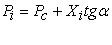Where(7)

Using of the equation (7) to interpolate the atmospheric pressure in the direction vectorfor the billing month accept February 2012, while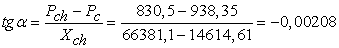From the equation (7) In case of Xi =0→Pi=Pc and in Xi =Xchim→Pi=Pchim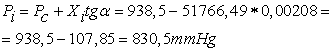Example, Xi =0,5Xch=0,5х51766,49=25883,245mNow through the magnitude of the vector (gradient) find the coordinates of the point i, relative to which the interpolated value of the atmospheric pressure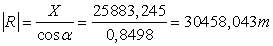, thenFor the interpolated value of the atmospheric pressure in the basin Chirchik point i with Pi = 884,479 mm. Hg. Article coordinates are: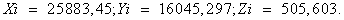Thus, when calculating compliance with the above rules interpolated value of the atmospheric pressure on the space of any territory, ask any point through the origin and can be found by analytical values and coordinates interpolated climate data.

To confirm the applicability of the proposed method for other climatic parameters interpolation to produce humidity. Calculation algorithm similar to the calculation for the atmospheric pressure:

The equation for interpolation humidity: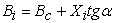(8)Verification of applicability of (8) in Xi = 0→Bi=Bc =72,25% and in Xi =Xchim.

Set the desired point interpolation: Xi =0,5Xchim=0,5х55947,77=27973,885m then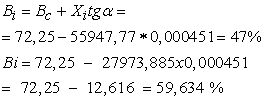Calculation of vector modules to establish the coordinates of the desired point-i make payment module gradient (the coordinates, length of the line on which the changes in humidity) to meteorological station Chimghan using the calculated values for the month of February Bch (55947,77; 35110,47; 1089,82).Calculates the cos of angle between the vector (gradient) and its projections on the coordinate axes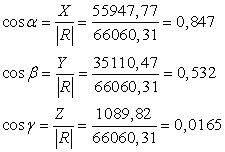Establish coordinates interpolated point on the site. Using the magnitude of the vector (gradient) find the coordinates of the point i, relative to which the interpolated values of humidity.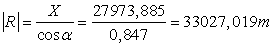, then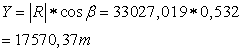Interpolated value for humidity in the basin Chirchik point i with Вi = 59.634% and the coordinates are: Xi = 27973,885; Yi = 17570,3; Zi = 544,95.

Thus, interpolation is performed other climatic factors. It is proposed to use thealgorithm of calculation (Figure 6).

### 3. Conclusion

Note, interpolation kriging and co-kriging deserve attention, so using them can interpolate any data concerning the territory. Nevertheless, for the use of the kriging methods and co-kriging is necessary to create a variogram or semi-variogram, and they require a model landscape and its mathematical formulation. What makes them effective for the interpolation of climate data in the decision less complicated engineering tasks scheduling irrigation of arid lands.

Methods inverse distance weighted (WRA) and the method of trend surface almost in different variations are used to interpolate the climate of these areas, but they give large errors in the interpolation of data on the transition zones with arid climate areas. So the transition from the mountain to the foothills and the plains affect sensitive to changing climatic parameters, and these methods can not take into account peculiarities of the transition zones with arid conditions of the area.

Binding interpolation to one reference point of measurement in all existing interpolation methods, in particular methods of Kriging, co-kriging methods of surface trend make them less accurate than when the reference point is the centre of the system. So centre where climatic parameter is the average of the total number of measurements is known purity parameter depends on the number of measured data.

Proposed method of interpolation with reference to the central reference point and using known and proven approaches graphoanalitical simplify the calculation process and eliminate errors in determining the estimated climatic parameters territory. Above algorithm allows creating a simple computer program for the production of the proposed interpolation method.

### Acknowledgement

I would like to express my sincere gratitude to my supervisor Professor Yasuhiro MITANI for his time, patience, advice, encouragement and continual support. I also express my respect and gratitude to Professor Tetsuya KUSUDA for valuable suggestion and comments for improvement of this scientific research paper.

### References

  I. Blyutgen, Geography climates, 2nd ed. Moscow, Russia: Progress, 1973, p. 402In article  M. A. German, Space methods of research in meteorology. Leningrad, Russia: Gidrometeoizdat, 1985, p. 310In article  V.F. Zhuravlev, Foundations of Theoretical Mechanics, 2nd ed. Moscow. Russia: FIZMATLIT, 2001, p 23.In article  M. Valipour, "Comparative Evaluation of Radiation-Based Methods for Estimation of Potential Evapotranspiration." J. Hydrol. Eng., 04014068., Sept.19, 2014.In article  Phillips N.A., A coordinate system having some special advantages for numerical forecasting, J.Meteorol, 1957.-N14.-PP.J. Padhye.In article  M. Valipour,“Analysis of potential evapotranspiration using limited weather data”, J. Applied Water Science, Springer Berlin Heidelberg, Sept.27, 2014.In article  E. N. Lorenz, Nature and the theory of the general circulation of the atmosphere. Leningrad, Russia: Gidrometeoizdat, 1970, p. 259.In article  "Atmosphere". Directory. Leningrad, Russia: Gidrometeoizdat, 1991, p. 510.In article  V. Petrov, H. Egamberdiev, B. Kholmatzhonov, T. Alaudinov. Meteorology. Tashkent, Uzbekistan: NUU, 2006, p. 330.In article  L.A. Khandozhko, Meteorological Service of the economy. Leningrad, Russia: Gidrometeoizdat, 1981, p. 232.In article  E. I. Zielinski, Data interpolation methods. Dnepropetrovsk, Ukraine: National Mining University, 1998.In article PubMed  Zhuravlev VF Foundations of theoretical mechanics. 2nd ed. - M.: Fizmatlit, 2001. - 320.In article